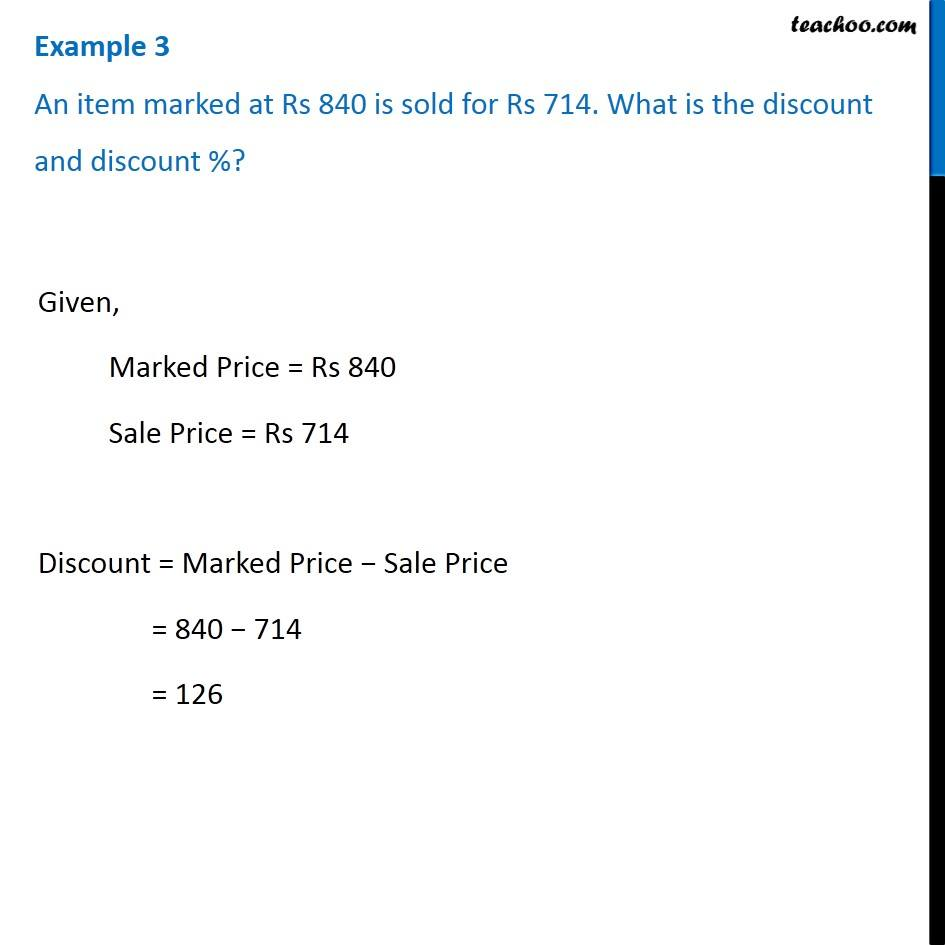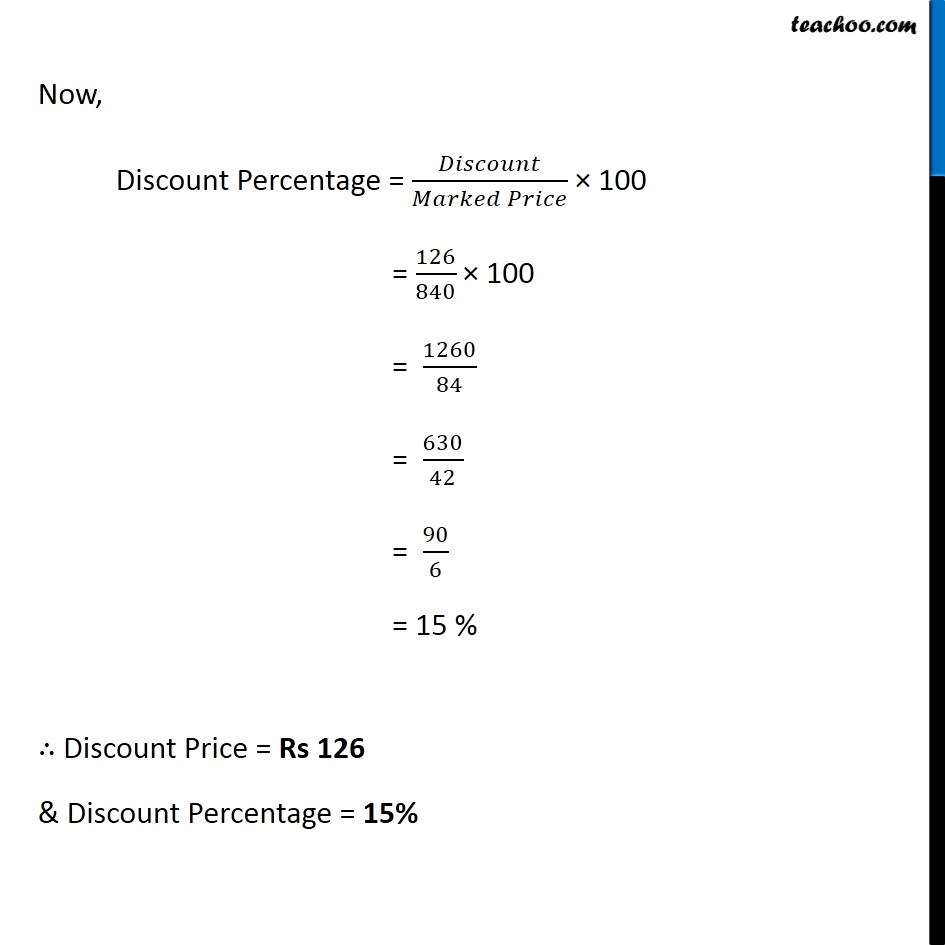1. Chapter 8 Class 8 Comparing Quantities
2. Concept wise
3. Finding Discounts

Transcript

Example 3 An item marked at Rs 840 is sold for Rs 714. What is the discount and discount %?Given, Marked Price = Rs 840 Sale Price = Rs 714 Discount = Marked Price − Sale Price = 840 − 714 = 126 Now, Discount Percentage = 𝐷𝑖𝑠𝑐𝑜𝑢𝑛𝑡/(𝑀𝑎𝑟𝑘𝑒𝑑 𝑃𝑟𝑖𝑐𝑒) × 100 = 126/840 × 100 = 1260/84 = 630/42 = 90/6 = 15 % ∴ Discount Price = Rs 126 & Discount Percentage = 15%

Finding Discounts